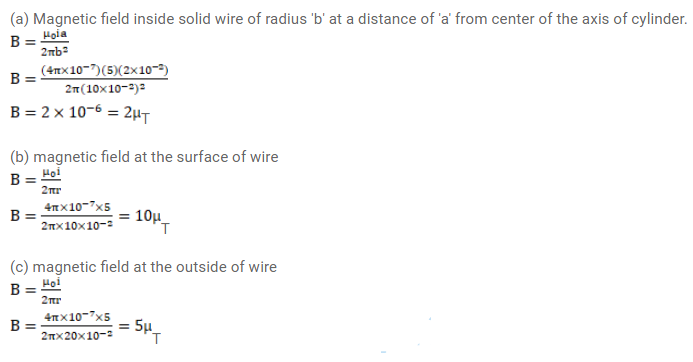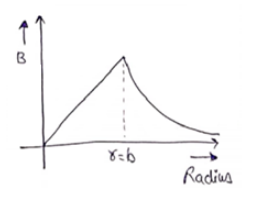# A solid wire of radius 10cm carries a current of 5.0A

Question:

A solid wire of radius $10 \mathrm{~cm}$ carries a current of $5.0 \mathrm{~A}$ distributed uniformly over its cross-section. Find the magnetic field $B$ at a point at a distance

(a) $2 \mathrm{~cm}$

(b) $10 \mathrm{~cm}$ and

(c) $20 \mathrm{~cm}$ away from the axis. Sketch a graph of $B$ versus $x$ for \$0

Solution: